## Sunday, February 26, 2012

### Infinity: Operations on Ordinals

This is the seventh post of the Infinity Series. For the first post, see here. For all posts, see the Infinity Series Portal.

In the previous post, the concept of ordinal numbers was introduced, as well as the idea of succession. Now, one can build the idea of ordinal addition by considering iterated succession. In the definition of ordinal addition, a recursive scheme is used, with succession as each step in the addition process.

The definition of addition is as follows for two (finite) ordinals α and β:
α + 0 = α
α + β' = (α + β)'

This procedure works for any finite ordinals (natural numbers). For example, the sum of 4 and 3 is

4+3 = 4+2' = (4+2)' = (4+1')' = ((4+1)')' = ((4+0')')' = (((4+0)')')') = (((4)')')' = ((4')')' = (5')' = 6' = 7

However, when attempting to evaluate the addition of infinite ordinals, one encounters a snag. Consider the ordinal ω, which is the set of all finite ordinals (natural numbers). For example, one cannot evaluate 1 + ω using the previous rule, as ω is not the successor of any ordinal, as it is the limit of the natural numbers. Ordinals which cannot be expressed as successors of others are accordingly known as limit ordinals.

The succession scheme above leads to all the familiar properties of addition for natural numbers, commutativity, associativity, and the like. However, the addition of infinite ordinals does not possess all of these properties. Consider the sum ω+1. The succession-based addition scheme works here, as

ω + 1 = ω' = ω∪{ω},

or, in more familiar set notation, the set of ordinals

ω + 1 = {0,1,2,3...,ω}, which is not equal to ω, as it has ω as a member, and no ordinal has itself as a member, only all previous ordinals. Therefore, unlike in cardinal addition, where adding any natural number to ℵ0 leaves it unchanged, ω + 1 is distinct from ω.

However, now consider 1 + ω. Although this expression cannot be evaluated using the previous method, one can intuitively think of the addition as the limit over natural numbers n of

1 + n,

which simply is, as n increases without bound among the natural numbers, ω. This is because the limit of a sequence of natural numbers can never exceed ω.

1 + ω = ω ≠ ω + 1

Paradoxically, these ordinals are different, but have the same cardinalities, namely ℵ0. To reinforce this fact, one can readily identify a one-to-one function f from ω + 1 to ω defined in the following way:

f(α) = α + 1, if α ≠ ω and f(α) = 0, if α = ω

Therefore, f(0) = 1, f(1) = 2, and so on, with each natural number being mapped to another, clearly within the range, ω, and the final member of ω + 1, ω, being mapped to 0. The methods used here are similar to those that were earlier used to demonstrate cardinal equalities.

In a similar fashion, 2 + ω = ω, and for that matter n + ω = ω for any natural number n. Also, ω + n > ω for any n ≥ 1, being instead equal to the set {0,1,2,3...,ω,ω + 1,ω + 2,...,ω + n - 1}

Ordinal multiplication can now be introduced as iterated addition, with

α*0 = 0
α*β' = α*β + α

Trivially, by this definition, ω*1 = ω*0 + ω = ω. Also, 1*ω is the same as the operation "+1" iterated for each natural number, i.e. an infinite number of times. In the same way as 1 + ω, 1*ω = ω.

However, what about ω*2? By the definition of multiplication it is equal to ω + ω. Clearly this is greater than ω and is equal to the set of ordinals {0,1,2,...,ω,ω + 1,ω + 2,...}. However, 2*ω is the limit of 2*n as n increases through the natural numbers. In the same way as 1 + ω, 2*ω = ω, hence ordinal multiplication is not commutative.

The above cases can be generalized to any ordinal of the form ω*m + n. Such an ordinal is strictly greater than ω, but is countable, i.e. there exists a bijection from this set to ω. Such a function is easily constructible along the lines of the linear function y = mx + n. To illustrate this process, consider the example ω*2 + 3 = {0,1,2,...,ω,ω + 1,ω + 2,...,ω*2,ω*2 + 1,ω*2 + 2,ω*2 + 3}. The function from this ordinal to ω is constructed as follows:

0 -> 3, 1 -> 5, 2 -> 7,... (finite ordinals α transform to α*2 + 3)
ω -> 4, ω + 1 -> 6, ω + 2 -> 8,... (ordinals of the form ω + α, for finite α, transform to α*2 + 4)
ω*2 -> 0, ω*2 + 1 -> 1, ω*2 + 2 -> 2

Clearly, this assigns a unique natural number (member of ω) to each element of the set ω*2 + 3, and the latter set is therefore countable, with a cardinality of ℵ0. All ordinals of the form ω*m + n have this same cardinality.

Finally, to extend the system of infinite ordinals further, one must introduce ordinal exponentiation, defined as follows:

α0 = 1
αβ' = (αβ)*α

For limit ordinals, the same limit argument used before will apply. Using these three operations, together with the idea of a limit, one can define many other infinite countable ordinals, a topic explored in the next post.

Sources: Axiomatic Set Theory by Patrick Suppes, http://en.wikipedia.org/wiki/Ordinal_addition

## Saturday, February 18, 2012

### Infinity: Ordinal Numbers

This is the sixth post in the Infinity Series, the first of which is found here. For all posts, see the Infinity Series Portal.

In order to further explore the concept of infinity, one must temporarily move away from the concept of the cardinal number, and consider yet another type of number: the ordinal. The system of ordinal numbers is again entwined in set theory, and, unlike cardinal numbers, they can be expressed as sets. It is also important to know that any cardinal number can alternatively describe a family of sets, i.e. all of the sets that have that cardinality. For example, the cardinal number 3 not only describes a number of members that a set can have, but also represents the family of all sets with three members.

Formally, ordinal numbers are very similar to cardinal numbers in that, in finite situations, they represent properties of classes of sets. The property that an ordinal number represents is known as order type. However, the class of sets whose order type is described by an ordinal number much more specific than that of a cardinal number. To understand the type of set that has an order type can represent, one must first understand the concept of a well-ordered set.

A well-ordered set is a set that can be well-ordered by a relation. A relation, in this case, is just a quantity that connects pairs of elements. A good example is the relation "<" or "less than". To understand well-ordering, observe the example below.

Consider the set S = {2,5,7}, and the relation R = <
If "<" is restricted to the set S, it simply means that the two elements of each ordered pair of the relation must come from S, i.e. chosen from 2, 5, or 7. Of the six possible ordered pairs, (2,5), (2,7), (5,7), (5,2), (7,2), and (7,5), three are members of the relation <, namely (2,5), (2,7), and (5,7). This is because 2<5, 2<7, and 5<7. Obviously, the remaining pairs are not, as 5 is not less than 2, and so on.

In this case, "<" well-orders S, meaning that each (non-empty) subset of S has a "minimal" element. For the relation "<", the "minimal" element of a subset is simply its smallest member. Since it is easy to find the smallest number of any subset of S, it is a well-ordered set.

In contrast, consider the set of all whole numbers N = {1,2,3...}. The above relation "<" does well-order the set, because, for any subset, one can state the smallest element. However, this does not hold with the operation "greater than" or ">". For example, if the subset N' is the set of natural numbers greater than 3, i.e. {4,5,6...}, then there is no "minimal" element under the relation ">", as there is no element in the set that is greater than all the others. However, overall, the set is still "well-ordered", because there is at least one relation that does satisfy the needed conditions.

Now, returning to the class of sets a certain order type, consider all the well-ordered sets that can be put into one-to-one correspondence (i.e. all the well-ordered sets with a given cardinality). However, this correspondence is of a rather special type, matching up the "minimal" elements with each other, followed by the "next-to-minimal" elements, and so on. Every class of sets for which all the members are connected is an equivalence class, and all sets in this class have an identical order type, which is a certain ordinal number.

For instance, the above set S = {2,5,7} under "<", and the set T = {b,c,d} under the relation that will be defined as "alphabet" (which puts alphabet in order), can be related by a correspondence. The minimal element of set S under "<" is clearly 2, and the minimum of T under "alphabet" is b, with b being the letter in the set closest to the beginning of the alphabet. Since the other elements can be paired in a similar way, these two sets are therefore members of an equivalence class and are of the same order type (intuitively, they are this order type because they each have three elements).

However, for each equivalence class, it is convenient to chose one, and only one set to be representative of the entire class, and thus, the ordinal. The first such set is the empty set, Ø, having no elements and being the only member of its equivalence class. We identify this set with the ordinal number "0". Therefore,

0 = Ø = {}

Hence forth, the representative set of each equivalence class is defined as the set containing all of the previous representative sets. For example, the successor of 0 is denoted "1", or the second ordinal number, and is defined as

1 = {Ø}

Note that this set is not the empty set, but rather the set containing only the empty set. It is obvious that this set can be well-ordered, as, trivially, there is only one element to order. It is equally clear that this set is representative of the class of sets with a single element and that it can be put in a one-to-one correspondence with each set in that class. Continuing the pattern of each representative set containing all of those previously, we have

2 = {Ø, {Ø}},
3 = {Ø, {Ø}, {Ø, {Ø}}},
and so on. Note that "2", has 0 and 1 as members, and "3" has 0, 1, and 2 as members.

Henceforth, "ordinal number" will refer to a set of this type, and 0, 1, 2, 3,... will refer to ordinal numbers unless otherwise specified. These ordinal numbers are well-ordered by the membership relation, or the relation set up between two sets with the second containing the first. In other words, each element of an ordinal number set is a member of all subsequent elements in the set. For example,

4 = {Ø, {Ø}, {Ø, {Ø}}, {Ø, {Ø}, {Ø, {Ø}}}}

In this set, each member is a member of each subsequent member, namely, Ø, being the first element of 4, is a member of {Ø}, {Ø, {Ø}}, and {Ø}, {Ø, {Ø}}}, which, of course, are just the ordinal numbers 1, 2, and 3. The minimal element for each ordinal number is Ø.

It is clear that one can go on to define any natural number as a set of this type, namely as the set of all previous ordinals. As with cardinal numbers, the first infinite ordinal number corresponds to the set of all natural numbers. This ordinal is denoted ω, and has every (ordinal) natural number as a member. It is clear that this set can be put in a one-to-one correspondence with the set of "regular" natural numbers, and it therefore has a cardinality of aleph-zero. However, the world of infinite ordinals is very different than that of infinite cardinals.

One crucial difference is the idea of succession in ordinals. From any ordinal α (greek letters are often used for ordinal variables), there is a successor to α, denoted α', which is defined as the next ordinal after α. Formally, α' is the smallest ordinal which has α as a member. Furthermore, α' can be constructed using the formula

α' = α ∪ {α}

where ∪ is the symbol for a union of sets. The concept of union entails combining all the members of two sets into one, and deleting any duplicates. For instance,

{1,4,6} ∪ {3,6,13} = {1,4,6,3,6,13} => {1,4,6,3,13}

Returning to the succession formula above, note the distinction between α and {α}. The first is an ordinal, but the second is not, being rather a set containing a single ordinal, α, as its only member. To more easily visualize this process, consider the simplest example:

0 = Ø
0' = Ø ∪ {Ø}

Now, the union of the empty set with any other set is simply the other set, as the empty set contributes no members to the result. Therefore,

0' = Ø ∪ {Ø} = {Ø},

the final term of which is, in fact, the ordinal 1! Though ordinal addition has not been defined, we can observe that the succession operation, in a more general case, identifies with the process of adding 1 (0+1=1 and 0'=1, etc.). The role that succession plays in infinite ordinals, as well as in operations on ordinals, is considered in the next post.

Sources: Axiomatic Set Theory by Patrick Suppes

## Friday, February 10, 2012

### Infinity: Uncountable Sets

This is the fifth post of the Infinity Series, beginning here. For all posts, see the Infinity Series Portal.

In previous posts, the idea of an "uncountable set" has arisen, namely a set that has a cardinality greater than that of the natural numbers. We have already revealed that the sets of real numbers and complex numbers are uncountable, specifically having a cardinality that is two to the power of aleph-zero, the cardinality of the natural numbers. However, there are other sets which have this cardinality, as well as sets with one greater than this.

First, sets with the cardinality of the continuum will be listed. The sets of real and complex numbers fit into this category, along with any interval of either of them. For example, the set of real numbers between 1 and 5 (inclusive or exclusive) will have the cardinality of the continuum.

A somewhat more interesting case is the Cantor set, a famous entity in mathematics. It is obtained geometrically by beginning with a solid line segment, (mathematically, this segment is the real numbers on [0,1]) and on each step removing the middle third of every existing line segment. This process is illustrated below.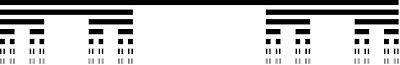The first seven steps in producing the Cantor Set. This process is repeated infinitely many times. It appears that the entire original line segment will eventually be removed after an infinite number of the above steps, and this is, in a way, true. During the first step 1/3 of the total bar is removed, followed by 1/9 of the total for each remaining segment on the second step, then 1/27 of the total for each remaining part on the third step, and so on. The sum of these removals is 1/3+2/9+4/27+... since there are 2^n segments on the nth step (the amount removed on second step equals 2*1/9=2/9, and on the third, 4*1/27=4/27). The above sequence sums, in the limit as n increases without bound, to 1. In other words, 100% of the original segment is taken away as the Cantor Set is constructed.

Despite the apparent paradox, it is clear that for any segment remaining at any step of the above process, it will have two endpoints that are untouched even after an infinite number of steps. This is because only the middle of all such segments are removed. The remaining points, which all become endpoints at some step, are the points of the Cantor Set. For example, the points 0 and 1 are never removed, along with 1/3, 2/3, 1/9, 2/9, 7/9, 8/9 and many others. The cardinality of this set can be discovered by expressing all its points in base-3 (ternary) decimal notation. In it, 1/3=.1=.02222..., 2/3=.2=.12222..., 1=.22222..., 7/9=.20222..., etc. Many of these numbers have two forms, i.e. 1/3=.1=.0222..., but it can be shown that the Cantor Set contains only points whose base-3 decimal representation has at least one form (out of the two) that consists of only 2's and 0's (a more full discussion is found here). If one took all of these decimals and changed all of the 2's into 1's, a set of binary sequences would be produced. The Cantor set has all base-3 points consisting of only 2's and 0's, but after the function, which is clearly a bijection, it is now the set of all binary sequences, which has previously been shown to have the cardinality of the continuum. The Cantor Set does as well.

Mathematically, this is remarkable. Even though the Cantor Set is an infinitesimal fraction of the line segment [0,1], it still has the cardinality of the continuum. Since no two points of the final Cantor Set are "adjacent" to each other, the Cantor Set is defined to have the property of being nowhere dense. Simply put, this means that any interval containing two points of the Cantor Set does not necessarily contain a third. In contrast, there are an infinite number of rational numbers between any two one could chose, and the set of rational numbers is therefore dense. The fact that such a "nowhere dense" set can have the cardinality of the continuum is also incredible, because all of the discrete sets (natural numbers, integers, etc.) that we have examined have not had this cardinality. These are clearly nowhere dense, as there are real numbers between any two natural numbers, or any two integers.

Another very interesting case is the cardinality of the set of continuous functions on the real numbers. To find this cardinality, it is useful to use a proof of trichotomous exclusion. Such a proof confirms that a set must have a cardinality greater than or equal to that of a certain set, and then proves that it must simultaneously satisfy the condition of having a cardinality less than or equal to that of the same set. Since one quantity cannot be less than and greater than another, the cardinality of the two sets must be equal.

To go about the above proof, it is useful to reevaluate the definition of a continuous function. The "local" definition is of most use here. It states that for any value x on a continuous function, there exists a sufficiently small ε such that f(x+ε) approximates f(x) to an arbitrary accuracy σ. In addition, as ε goes to 0, σ will as well, as point Q becomes a better and better approximation to point P. This is all summarized in the figure below.Compare the above to the discontinuous function below, where the open circle on the curve represents a discontinuity, the true function value f(x) being located at point P. Even with ε miniscule, the approximation to f(x) never reaches the desired accuracy of σ.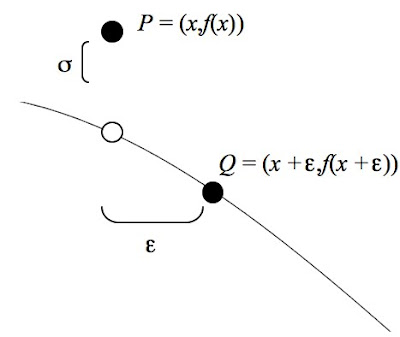Having defined what it means to be continuous, it is now possible to find the cardinality of the set of continuous functions. The key concept is that knowing the value of a continuous function on all rational numbers is enough to determine it uniquely, i.e. to find its value at any real number.

To see this, consider the value x from above to be a certain real number (for our purpose, make it irrational). This number can be approximated to an arbitrarily high accuracy with a rational number, e.g. π≈3.14159=314159/10000, etc. and therefore ε can be made as small as desired. In the limit as ε goes to 0, the approximation to f(x) becomes exact, and the function value is uniquely determined. Therefore, the knowledge of the value of a continuous function over the rational numbers is enough to determine it.

The main idea is that the above shows that the any specific continuous function needs no more than a countable set of real numbers to define it. The cardinality of the set of all continuous functions is therefore either equal to or less than that of the set of the countable sets of real numbers, or the power set of real numbers, (a power set is the set of all combinations of elements of a given set, discussed in an earlier post) whose cardinality is 2 to the power of the cardinality of all countable sets: aleph-zero. The set of continuous functions therefore has no more than the cardinality of the continuum.

To confirm that this is the cardinality, we must still prove that the set of continuous functions is uncountable. There is a very simple way of doing this. Take a subset of the continuous functions, the constant functions, for which f(x)=k, k being a real number. Clearly, if one defines a set of all constant functions, including every possibility of k, the set will be equivalent in cardinality to the real numbers: the cardinality of the continuum. However, the constant functions are a subset of all continuous functions, the true cardinality must be equal to or greater than this. Since we have already confirmed that it is no greater, and now it is known that the cardinality of the set is no less than that of the real numbers, it follows that the two must be equal.

In contrast, discontinuous functions cannot be pinned down without their value at each and every real number. For example, the discontinuous (piecewise) function that defines f(x) to be equal to 1 for all x except π, and at π for the value to be 12, no degree of accuracy in the rational numbers can confirm that f(π) will equal twelve. The best approximations will simply yield f(π)=1, as they "expect" the function to be continuous at π. More generally, some discontinuous function could potentially have a jump at any or every real number. Therefore, it takes a set of real numbers to uniquely determine such a function.

The total set of functions (including both continuous and discontinuous) on the real numbers is then the power set of the real numbers, with a cardinality greater than any yet discussed. It is 2 to the power of the cardinality of the continuum, which is also known as beth-two, the symbol of which is illustrated below.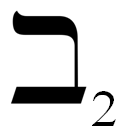This is the third member of what is called the beth sequence. Beth-zero is equal to aleph-zero, and each subsequent element of the sequence is defined recursively as 2 to the power of the previous one. Beth-one is equal to 2 to the power of aleph-zero, or the cardinality of the continuum, and so on. Equivalently, each element is the cardinality of the power set of the previous element. The distinctions between the aleph series and the beth series are revealed in a later post. The next post is on a new type of number.

Sources: http://en.wikipedia.org/wiki/Cantor_set, etc.

## Thursday, February 2, 2012

### Infinity: Operations on Cardinals

Before reading this post, make sure you have read the first three parts of the Infinity Series, the first of which is found here. For all posts, see the Infinity Series Portal.

Having found the mathematical relationship between aleph-zero and the cardinality of the continuum, one wonders if it is possible to perform other operations with infinity cardinals, and whether these equations create any numbers not yet discussed. To start, take a simple addition from cardinal arithmetic:
(1)

What exactly does this equation mean? We are asked to "add" two quantities, one of which is an infinite cardinal, and one is a simple number. That it is possible to evaluate the sum (1) follows from the fact that aleph-zero and 1 are both cardinal numbers. Since they are of the same number system, they are "compatible" in a way, and can be combined by the use of sets. We have already proved (1) in the first post of the series, but let us recap. It has been discussed that adding the element 0 to the set of natural numbers does not change its cardinality, since the function y=x-1 from one set to the other is a bijection. In set notation, with vertical lines representing cardinality: |{0}|+|{1,2,3...}|=|{0,1,2,3...}| Note that if one replaces the values in this equation with the actual cardinalities, one obtains the equation (1) above! The equation in simple sets that has the same meaning as a cardinal equation will henceforth be known as the Corresponding Set Equality (CSE).

The generalization of (1) for any natural number n is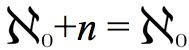(2)

This can also be transformed into a CSE, since any subset of integers also has cardinality aleph-zero. The the set {-n+1,-n+2,...-2,-1,0} clearly has n elements, and can be added to the natural numbers to form the CSE, namely: |{-n+1,-n+2,...-2,-1,0}|+|{1,2,3...}|=|{-n+1,-n+2,...-2,-1,0,1,2,3...}|. By evaluating the cardinalities on both sides, one obtains equation (2). It is easy to continue on to other arithmetic operations, such as multiplication: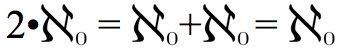(3)

This particular equation also has a CSE. First consider the set of integers. It is clear that it can be split into two components, both of which have a cardinality of aleph-zero, namely the whole numbers: {0,1,2,3...} and the negative integers: {...-3,-2,-1} However, when these two sets are combined, the set of integers results, which we already know has cardinality aleph-zero as well. The CSE here is |{0,1,2,3...}|+|{-1,-2,-3...}|=|{...-3,-2,-1,0,1,2,3...}|. All three of the cardinalities are evaluated as aleph-zero, and (3) results. Now we shall prove the general multiplication result(4)

for any natural number n. We have already determined that the rational numbers, and any infinite subset of them have cardinality aleph-zero. Consider the set {a/n,1+(a/n),2+(a/n),...}. This is simply the set of natural numbers with the rational number a/n added to each term. For a constant n, one could consider creating a set for each integral value of a from 0, to n-1, inclusive. This produces n sets of cardinality aleph-zero.

An example will make this more clear. Consider the case with n=3. For a=0, the set produced is simply the whole numbers ({0/3,1+(0/3),2+(0/3)...}={0,1,2...}) For a=1, the set is {1/3,1+(1/3),2+(1/3)...} and for a=2, the set is {2/3,1+(2/3),2+(2/3)...}, all of which have cardinality aleph-zero. The sum of these sets is {0,1/3,2/3,1,1+(1/3),1+(2/3),2...}, or the set of all multiples of 1/3, which also has the same cardinality. We have just proved (4) for n=3. The more general CSE for (4) is |{0/n,1+(0/n),2+(0/n)...}|+|{1/n,1+(1/n),2+(1/n)...}|+
|{2/n,1+(2/n),2+(2/n)...}|+...+|{(n-1)/n,1+((n-1)/n),2+((n-1)/n)...}|=
|{0,1/n,2/n...,1,1+(1/n),1+(2/n),...}|, the final set being the set of multiples of 1/n.

This equation is tedious, but it simply is the division of the set of multiples of 1/n into n parts, all of which have cardinality of aleph-zero. Since the set of multiples on the right hand side of the equation as an equivalent cardinality, this proves (4). Moving on, even the multiplication of two infinite quantities is possible.(5)

The CSE for this equation follows from the countability of the set of ordered pairs. The set of ordered pairs (x,y) with natural numbers x and y can be split into components by setting a value for x, for example 1, and letting y vary among the natural numbers. For each constant value of x, a set of cardinality aleph-zero is generated, and since there are aleph-zero choices for x, the above result (5) follows. In CSE form, |{(1,1),(1,2),(1,3)...}|+|{(2,1),(2,2),(2,3)...}|+...=[the cardinality of the set of ordered pairs]. Each quantity in the equality has value aleph-zero when evaluated, and since there are as many members on the left side, it follows that the cardinality of the natural numbers, when multiplied by itself, yields the same quantity. This result can too be generalized to any positive integer power: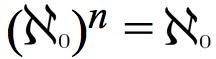(6)

Since the case n=2 makes use of ordered pairs, it is natural to assume that higher powers will involve the corresponding ordered n-tuplet. This is correct. There are aleph-zero possibilities for each element of an integral ordered n-tuplet, and each choice of element contributes an aleph-zero to the product. The end result is aleph-zero to the nth power, but since it has already been said that the cardinalities of the sets of ordered n-tuplets for finite n are all aleph-zero, the equality (6) is a direct result.

Summarizing the above, no additions, multiplications, or nth powers, when applied to aleph-zero, change its value. However, it has already been shown that two taken to the power of aleph-zero produces a different infinite cardinal, namely the cardinality of the continuum. But what about a general natural number n taken to the same power, or even aleph-zero taken to the power of itself?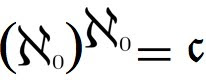(7)

Remarkably, we find that this quantity is equal to the cardinality of the continuum! This can be derived intuitively from the result (6). Since aleph-zero to the power of n is equal to the cardinality of the set of the ordered n-tuplets, one obtains (7) by letting n increase without bound to aleph-zero, at which point one obtains the cardinality of the set of infinite sequences, which has previously been shown to be greater than aleph-zero, and named the cardinality of the continuum. Any other n taken to the aleph-zero power is also equal to the cardinality of the continuum, as such quantities would clearly be greater than 2 to that power and less than the left side of (7). Since these both have the same value, those of the general case do as well. This is all summarized below.The next post explores uncountable sets, namely stating what other sets besides the real or complex numbers have a cardinality equal to, or even greater than, the cardinality of the continuum.

Sources: http://en.wikipedia.org/wiki/Power_set